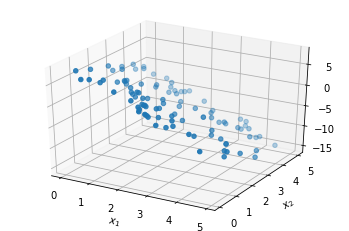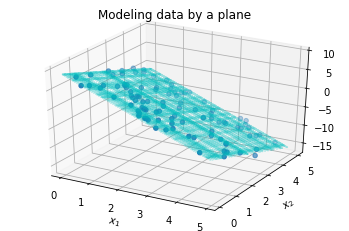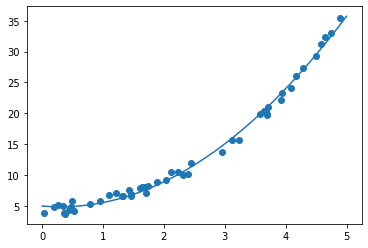# Supervised learning by regression¶

May 21, 2020

Machine learning refers to mathematical and statistical techniques to build models of data. A program is said to learn from data when it chooses a model or adapts tunable model parameters to observed data. In broad strokes, machine learning techniques may be divided as follows:

• Supervised learning: Models that can predict labels based on labeled training data

• Classification: Models that predict labels as two or more discrete categories
• Regression: Models that predict continuous labels
• Unsupervised learning: Models that identify structure in unlabeled data

• Clustering: Models that detect and identify distinct groups in the data
• Dimensionality reduction: Models that identify lower-dimensional structure in higher-dimensional data

In this activity, we focus on supervised learning. Note the two further subdivisions mentioned above within the category of supervised learning, and here are two examples within each for further orientation:

• Classification example: identify an email as spam or not (discrete label) based on counts of trigger words.
• Regression example: predict the arrival time (continuous label) of a streetcar at a station based on past data.

We shall further focus on regression in this activity. Regression addresses an age-old fitting problem: given a set of data, find a line (or a curve, or a surface, or a hypersurface in higher dimensions) that approximately fits the data. The equation of the line, in the machine learning language, is the model of the data that has been "learnt." The model can then "predict" the values, i.e., "labels" at points not covered by the original data set. Finding equations of curves that pass through a given set of points is the problem of interpolation, which goes at least as far back as Newton (1675). The fitting problem in regression, also known at least as far back as Gauss (1809), is a relaxed version of the interpolation problem in that it does not require the curves to pass through the given data, and is generally more suitable to handle noisy data. These days, when machine learning comes at you with the brashness of an overachieving new kid on the block, it is not fashionable to view the subject from the perspective of established mathematical techniques with rich histories. Instead, it has somehow become more fashionable to view machine learning as some sort of new AI miracle. Please do not expect any miracles here.

## Linear Regression¶

Let's start from the linear regression in a form you have seen previously: given data points $(x_i, f_i)$, $i=0,1, \ldots, N$, fit a linear equation $$f(x) = a_0 + a_1 x$$ to the data, in such a way that the error in the fit $$e = \sum_{i=0}^N | f(x_i) - f_i|^2$$ is minimized. Since the quantity on the right is a sum of squares, this is called the least-squares error. It is easy to solve this minimization problem. Writing

$$Y^\text{data} = \begin{bmatrix} f_0 \\ \vdots \\ f_N \end{bmatrix}, \qquad\quad Y^\text{fit} = \begin{bmatrix} f(x_0) \\ \vdots \\ f (x_N) \end{bmatrix} = \underbrace{ \begin{bmatrix} 1 & x_0 \\ \vdots & \vdots \\ 1 & x_N \end{bmatrix} }_{X} \underbrace{ \begin{bmatrix} a_0 \\ a_1 \end{bmatrix} }_{a}$$

the error $e$ can also be expressed as $e = \| Y^\text{fit} - Y^{\text{data}} \|^2 = \| X a - Y^{\text{data}} \|^2 = (X a - Y^{\text{data}})^t (X a - Y^{\text{data}}).$ Now, either from linear algebra, or from calculus, one concludes that $e$ is minimized when

$$a = (X^t X)^{-1} X^t Y^\text{data}.$$

This is the least-squares solution to the linear regression problem.

In the machine learning language,

• $f_i$ are (continuous) "labels",
• the "model" is the linear formula $a_0 + a_1 x$,
• the "labeled training data" is $(x_i, f_i)$, and
• the "predictions" are values of $f(x)$ at various $x$-values.

Here is an example.

In :
import numpy as np
from numpy.linalg import inv
%matplotlib inline
import matplotlib.pyplot as plt
rng = np.random.default_rng(123)

In :
x = 5 * rng.random(20)
f = 3 * x + 5 * rng.random(20)
plt.scatter(x, f); plt.xlabel('x'); plt.ylabel('Continuous labels (f)');The data has a linear trend, so let's try to fit a line to it using linear regression formula we derived above.

In :
X = np.array([np.ones(len(x)), x]).T
a = inv(X.T @ X) @ X.T @ f               # Create the "model"

In :
x_predict = np.linspace(0, 5, num=100)
f_predict = a  + a * x_predict     # "Predict" using the model

In :
plt.scatter(x, f)
plt.xlabel('x'); plt.ylabel('Continuous labels (f)');
plt.plot(x_predict, f_predict, 'c');To have a visual representation of the error that is minimized by this line, we can plot line segments (the red thick lines below) whose sum of squared lengths is what we minimized:

In :
from matplotlib.collections import LineCollection
fp = X @ a
plt.scatter(x, f)
lc = LineCollection([[(x[i], f[i]), (x[i], fp[i])]
for i in range(len(x))], color='r', linewidth=4, alpha=0.5)
plt.xlabel('x'); plt.ylabel('Continuous labels (f)');
plt.plot(x_predict, f_predict, 'c');Let us save there results for later comparison.

In :
linear_example = {'data': [x, f], 'model': a}


## Higher dimensions¶

The process of linear regression is very similar in higher dimensions. To fit some given data $f_i$ on $N+1$ points $\vec{x}_i$, each of which are $m$-dimensional, we express the points in coordinates $\vec{x}_i = (x_i^{(1)}, x_i^{(2)}, \ldots, x_i^{(m)})$. The model now is

$$f(x^{(1)}, x^{(2)}, \ldots, x^{(m)}) = a_0 + a_1 x^{(1)} + \cdots + a_m x^{(m)}.$$

Exactly the same algebra as before yields the same solution formula

$$a = (X^t X)^{-1} X^t Y^\text{data},$$

the only difference now being that $$a = \begin{bmatrix} a_0 \\ \vdots \\ a_m \end{bmatrix}, \qquad\quad X = \begin{bmatrix} 1 & x_0^{(1)} & x_0^{(2)} & \cdots & x_0^{(m)} \\ 1 & x_1^{(1)} & x_1^{(2)} & \cdots & x_1^{(m)} \\ \vdots & \vdots \\ 1 & x_N^{(1)} & x_N^{(2)} & \cdots & x_N^{(m)} \\ \end{bmatrix}$$ Here is a 3D example, where we can still attempt to visualize:

In :
x1 = 5 * rng.random(100)
x2 = 5 * rng.random(100)
f = 10 - (3*x1 + 2* x2 +  2 * rng.random(100))

In :
X = np.array([np.ones(len(x1)), x1, x2]).T
a = np.linalg.inv(X.T @ X) @ X.T @ f

In :
from mpl_toolkits.mplot3d import Axes3D
ax = plt.figure().gca( projection='3d')
ax.scatter(x1, x2, f)
ax.set_xlabel('$x_1$'); ax.set_ylabel('$x_2$');In :
ax = plt.figure().gca( projection='3d')
ax.set_xlabel('$x_1$'); ax.set_ylabel('$x_2$')
xx1, xx2 = np.meshgrid(x1, x2)
zz = a + a * xx1 + a * xx2
ax.plot_wireframe(xx1, xx2, zz, color='c', alpha=0.2)
ax.scatter(x1, x2, f); ax.set_title('Modeling data by a plane');We save these results for later examination.

In :
planar_example = {'data': [np.array([x1, x2]).T,  f], 'model': a}


## Curve fitting¶

If you know that your data is exponential, you might get better results by fitting with exponentials instead of linear functions. The "linear" regression process can be adapted to use exponentials, or gaussians, or indeed any basis functions you feel are particularly appropriate for your data set. The linearity in "linear" regression refers to the linear dependence of the model on the data (and has nothing to do which whether your model $f$ is linear or not).

Deriving the general formula is done by the same method. Using basis functions $\phi_j(\vec x)$, we can fit given data $f_i$ on $N+1$ points $\vec{x}_i$ using the model $$f(\vec x ) = a_0 \phi_0(\vec x) + a_1 \phi_1(\vec x ) + \cdots + a_m \phi_m(\vec x ).$$ Again, the previous algebra yields the same solution formula

$$a = (X^t X)^{-1} X^t Y^\text{data},$$

for the model parameters that provide the minimizer of $$e = \sum_{i=0}^N | f(\vec x_i) - f_i|^2.$$ The only difference now is that
$$a = \begin{bmatrix} a_0 \\ \vdots \\ a_m \end{bmatrix}, \qquad\quad X = \begin{bmatrix} \phi_0(\vec x_0) & \phi_1(\vec x_0) & \cdots & \phi_m(\vec x_0) \\ \phi_0(\vec x_1) & \phi_1(\vec x_1) & \cdots & \phi_m(\vec x_1) \\ \vdots & \vdots \\ \phi_0(\vec x_N) & \phi_1(\vec x_N) & \cdots & \phi_m(\vec x_N) \end{bmatrix}.$$

Here is an example where we fit a quadratic curve to a simple one-dimensional data set, i.e., here $$f(x) = a_0 + a_1 x + a_2 x^2$$ and the $a$'s are found by the above formula.

In :
x = 5 * rng.random(50)
f = 3 * np.exp(x/2) + 2 * rng.random(50)
plt.scatter(x, f); plt.xlabel('x'); plt.ylabel('Continuous labels (f)');In :
phi0 = np.ones(len(x))
phi1 = x
phi2 = x**2

X = np.array([phi0, phi1, phi2]).T
a = np.linalg.inv(X.T @ X) @ X.T @ f

In :
xcurve_predict = np.linspace(0, 5, num=500)
phi0 = np.ones(len(xcurve_predict))
phi1 = xcurve_predict
phi2 = xcurve_predict**2

fcurve_predict = a * phi0  + a * phi1  + a * phi2
plt.scatter(x, f)
plt.xlabel('x'); plt.ylabel('Continuous labels (f)');
plt.plot(xcurve_predict, fcurve_predict, 'c');If we had attempted to fit a straight-line through the data, then we would not have gotten such a close fit. Another way of saying this in the prevalent terminology is that linear features underfit this data, or that the linear model has high bias. Saving this example also for later, we continue.

In :
curve_example = {'data': [x, f], 'model': a, 'type': 'quadratic'}


## The module scikit-learn¶

All the regression computations we did above can be done using the module scikit-learn. Of course, the formulas above were simple and easy to implement. The power of scikit-learn is not in its linear regression implementation, but rather, in the vast range of many other ready-made facilities it provides under a unified user interface. When faced with a package that attempts to do so many things, it's a good entry strategy to confirm that it behaves as we expect in situations we know. This was our purpose in using the simple regression as an entry point into scikit-learn.

Let's check if our first-principles computation of regression solutions match what scikit-learn produces.

In :
from sklearn.linear_model import LinearRegression
model = LinearRegression(fit_intercept=True)


We can now fit data to this model using the fit method. Let's fit the same data we used in the first example of this activity.

In :
x, f = linear_example['data']   # Recall the saved data from the first example
model.fit(x[:, np.newaxis], f)  # Training step

Out:
LinearRegression(copy_X=True, fit_intercept=True, n_jobs=None, normalize=False)
In :
xfit = np.linspace(0, 5, num=100)
ffit = model.predict(xfit[:, np.newaxis])   # Prediction step
plt.scatter(x, f);
plt.xlabel('x'); plt.ylabel('Continuous labels (f)');
plt.plot(xfit, ffit, 'c');Clearly we seem to be getting the same result. We can confirm the results are exactly the same by digging into the solution components within the model object, as seen below. (Recall that in $f(x) = a_0 + a_1 x$, the coefficient $a_0$ is called the intercept.)

In :
model.intercept_, model.coef_

Out:
(2.9548137487468367, array([2.90310325]))

This is exactly the same as the values we solved for previously:

In :
linear_example['model']

Out:
array([2.95481375, 2.90310325])

#### Higher dimensions¶

The fitting process in scikit-learn is similar in higher dimensions.

In :
x12, f = planar_example['data']
model.fit(x12, f)

Out:
LinearRegression(copy_X=True, fit_intercept=True, n_jobs=None, normalize=False)
In :
model.intercept_, model.coef_

Out:
(9.167204926561409, array([-3.03592026, -2.03048875]))

This matches our previously computed results:

In :
planar_example['model']

Out:
array([ 9.16720493, -3.03592026, -2.03048875])

## More terminology¶

Of course, regression for curve fitting is also possible in scikit-learn. The difference now is that here you begin to see how things would get easier if you learn their language.

Scikit-learn uses the word estimator for models in machine learning. In the module, estimators are python objects that implement the methods fit and predict. We have already seen both methods in the context of the above regression examples. Additional terminology we should know include transformer (objects which can map/transform data into some other form) and pipeline (a sequence of transformers followed by an estimator).

The term feature is probably the most difficult one to pin down as it is used for too many things: data attributes, elements of a data row, columns of a data array, the range of a function mapping some data values, etc. When a data set is being fitted with some basis functions, linear or not, the word feature is used to refer to the basis. In fact, the process of selecting such basis functions is an example of feature engineering. More generally, feature engineering is any process by which raw information (data) is converted into numbers or other mathematical objects, things inside a feature matrix. Tidy data in a feature matrix has each variable/feature in a column and each observation/sample in a row.

In :
from sklearn.pipeline import make_pipeline
from sklearn.preprocessing import PolynomialFeatures


Using polynomial features, we create quadratic basis functions.

In :
q = PolynomialFeatures(3, include_bias=False)


Here is an example of a transform(er):

In :
data = np.array([5, 7, 9])[:, np.newaxis]
q.fit_transform(data)

Out:
array([[  5.,  25., 125.],
[  7.,  49., 343.],
[  9.,  81., 729.]])

As you can see the feature q performed the data transformation $$\begin{bmatrix} x_0 \\ x_1 \\ \vdots \\ x_N \end{bmatrix} \longrightarrow X = \begin{bmatrix} \phi_0(\vec x_0) & \phi_1(\vec x_0) & \cdots & \phi_m(\vec x_0) \\ \phi_0(\vec x_1) & \phi_1(\vec x_1) & \cdots & \phi_m(\vec x_1) \\ \vdots & \vdots \\ \phi_0(\vec x_N) & \phi_1(\vec x_N) & \cdots & \phi_m(\vec x_N) \end{bmatrix}$$ for $\{ \phi_i(x)\} = \{ x, x^2, x^3\}$.

#### Curve fitting¶

The point of view taken by scikit-learn for curve fitting is that it is a process obtained by applying the linear regression formula after applying the above transformer. Therefore, one can implement it using a pipeline object where this transformer is chained to a linear regression estimator. Here is how this idea plays out for the curve-fitting example we saw previously.

In :
x, y = curve_example['data']  # load data from the prior example

# make model/pipeline and fit the data to it:

Out:
Pipeline(memory=None,
steps=[('polynomialfeatures',
PolynomialFeatures(degree=2, include_bias=True,
interaction_only=False, order='C')),
('linearregression',
LinearRegression(copy_X=True, fit_intercept=True, n_jobs=None,
normalize=False))],
verbose=False)
In :
yfit = quadratic_model.predict(xfit[:, np.newaxis])
plt.scatter(x, y)
plt.plot(xfit, yfit);We can cross-check that the model parameters are exactly the same after fitting by examining the LinearRegression object in the quadratic model pipeline:

In :
quadratic_model.named_steps

Out:
{'polynomialfeatures': PolynomialFeatures(degree=2, include_bias=True, interaction_only=False,
order='C'),
'linearregression': LinearRegression(copy_X=True, fit_intercept=True, n_jobs=None, normalize=False)}
In :
quadratic_model.named_steps['linearregression'].intercept_

Out:
4.963796378670274
In :
quadratic_model.named_steps['linearregression'].coef_

Out:
array([ 0.        , -0.82755299,  1.39570211])

These match our previously computed results for quadratic fit:

In :
curve_example['model']  # previously saved results from first principles

Out:
array([ 4.96379638, -0.82755299,  1.39570211])

To conclude, we have built some confidence in scikit-learn's abilities under the hood. There is plenty of material online, including [JV-H], on how to use scikit-learn and other machine learning packages, and on important pitfalls such as overfitting. However, it may be a bit harder to find out the mathematics behind each software facility: the documentation is designed for quick users in a rapidly changing field, and therefore understandably does not get into the math. This may not be comforting to you as students of mathematics, so my focus here and in the next few lectures is to connect these software tools with the mathematics you know.